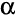# Overview of Statistical Testing in Q

 Project & table-specific Statistical Assumptions settings Q Rules that might override settings Question Type and merged values/categories in the Outputs Tab used for sample sizes and percentages Variable Type (Mean or Proportion testing) Value Attributes Table structure such as column spans Select significance test to run based on the data and settings Calculate p-values - The computed p-values for each cell can be shown by selecting p from Statistics - Cells. Correct p-values - If a Multiple Comparison Correction has been specified, the p-value is corrected to take this into account. When the False Discovery Rate Correction has been applied, which is the default, the Corrected p for each cell can be shown by selecting p from Statistics - Cells; with other types of Multiple Comparison Corrections then p itself is corrected. Identify significance - This is made by comparing p or Corrected p with the Overall significance level The result is displayed as being significant in accordance with the chosen method of display: Testing the cell complement: Font Colors: Red = lower Blue = higher Arrows: Arrow length = degree of significance Testing using Compare columns: Upper case = 99.9% confidence and higher Lower case = 95 - 99.9%

## Examples of when significance testing is conducted

The user can specify which relationships are to be tested by:

• Creating tables manually, by selection weights filters and questions from the blue and drop-down menus. Each cell in the table is then tested.
• Selecting individual cells on the table and pressing.
• Creating large batches of tables using Basic Tables. This is equivalent to creating tables manually, except that many are done at the same time.
• Creating batches of tables using Smart Tables. As with Basic Tables, this causes lots of tables to be created with significance tests conducted in each cell of the table. However, it also causes each table to be tested (which is equivalent to selecting all the cells in a table and pressing) and places the significant and insignificant tables in different groups.
• Regression. There are two quite different ways of doing this. It can be done by setting up an Experiment question (in which case each of the different ways of specifying relationships to test, as described in the previous bullet points, can be conducted), or, it can be done by selecting Create > Traditional Multivariate Statistics > Regression, in which case the p-values are reported (and the user is left to interpret results as being significant or not, based on the p-values or any other criteria that are considered applicable).
• Creating Segments, where for some models significance tests are presented for coefficients and information criteria, which are a type of significance test, are used to select the number of segments.